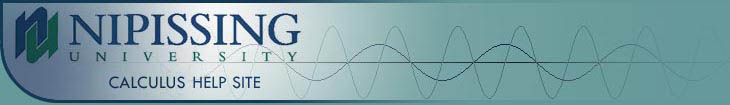TUTORIALS
TUTORIALS HOME

GENERAL MATH
NUMBER SETS
ABSOLUTE VALUE & INEQUALITIES
SETS & INTERVALS
FRACTIONS
POLYNOMIALS
LINEAR EQUATIONS
GEOMETRY
FINITE SERIES
TRIGONOMETRY
EXPONENTS
LOGARITHMS
INDUCTION

CALCULUS
LIMITS
DERIVATIVES
RELATED RATES & OPTIMIZATION
CURVE SKETCHING
INTEGRALS
AREA & VOLUME
INVERSE FUNCTIONS

MAIN
HOME
TESTS
TUTORIALS
SAMPLE PROBLEMS
COMMON MISTAKES
STUDY TIPS
GLOSSARY
CALCULUS APPLICATIONS
MATH HUMOUR

# Perimeters, Areas, and Volumes

Perimeter is the distance around a two dimensional (or flat) shape. Area (or surface area) is the amount of space a two dimensional surface occupies. In a three dimensional object, surface area is the area occupied by the outer surface of the shape. Volume is the amount of space a three dimensional object occupies. Here are formulas for the perimeters, areas, and volumes of some basic shapes as well as sketches of them.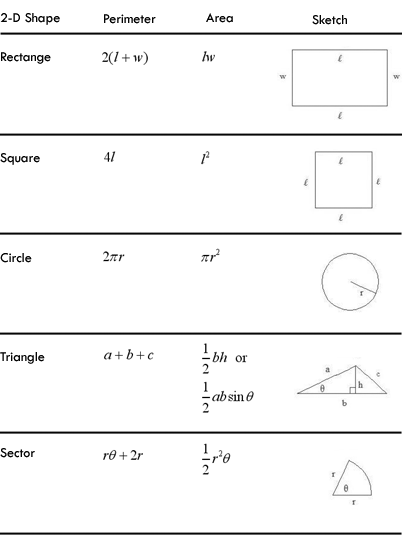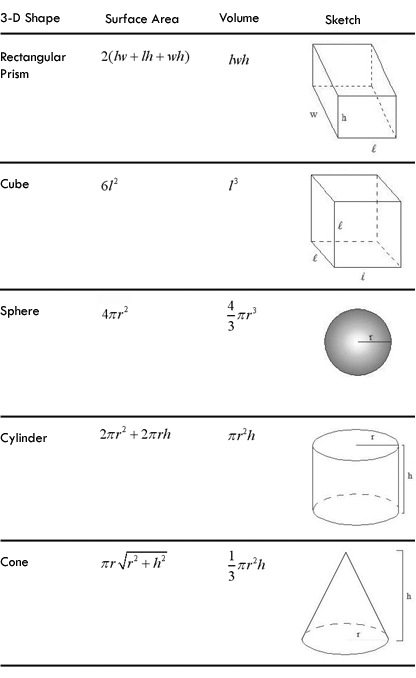# Examples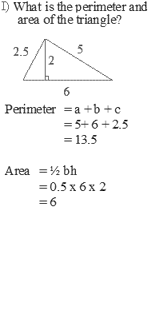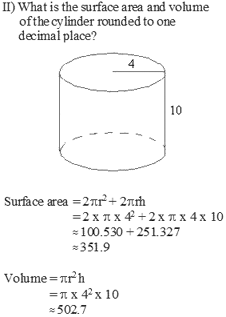Note: For another example of finding the volume and surface area of a figure, see question #1 in the Additional Examples section at the bottom of the page.

# Pythagoras Theorem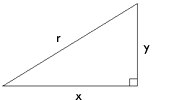The Pythagorean Theorem is used to calculate side lengths in right angled triangles. For hypotenuse r and sides x and y, the Pythagorean theorem states :

r2 = x2 + y2

Given any two side lengths, this formula can be used to calculate the third side.

# Examples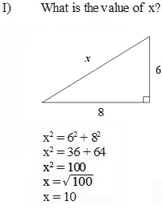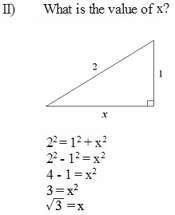# Distance and Midpoint of a Line Segment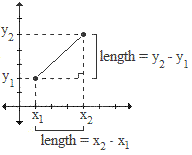The formula for the distance between two points (or length of a line segment) is derived from the Pythagorean Theorem. This is shown in the figure to the right and the derivation below.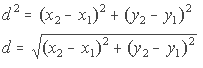In summary, the distance D, between any two points (x1, y1) and (x2, y2) is given by: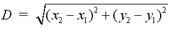The midpoint of a line segment is the point equidistant from both endpoints. If the endpoints of the line segment are (x1, y1) and (x2, y2) the midpoint is determined by the following formula: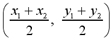The x and y coordinates of the midpoint are the averages of the x and y coordinates of the endpoints, respectively.

# Examples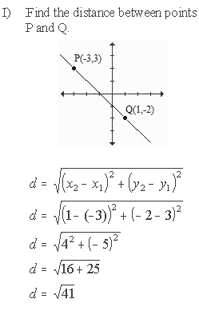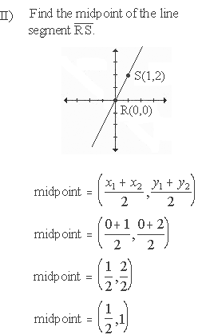Note: For another example of finding the midpoint and distance between two points, see question #2 in the Additional Examples section at the bottom of the page.

# Circle Equation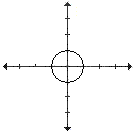A unit circle has it's center at the origin (0,0) and radius = 1. The general equation of the unit circle is x2 + y2 = 1. To change this circle's position or size, we use the following equation:

(x-h)2 + (y-k)2 = r2

where h is the factor by which the circle is shifted right or left, k the factor by which the circle is shifted up or down, and r the radius.

Note: In the formula, (x-h) will shift the circle to the right and (x+h) will shift the circle to the left. This is counter-intuitive from what would expect. Common sense would tell us that (x+h) would mean a shift to the right and (x-h) would shift to the left. This is not the case, however, so watch for this error when working with circles. Similarly, (y-k) shifts the circle upwards while (y+k) shifts the circle downwards. In addition, remember that in the formula, the radius r is squared. So a circle with equation x2 + y2 = 4 has a radius of 2 and not a radius of 4.

# Examples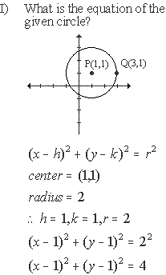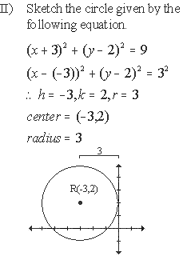# Other Conics

A conic is a shape which is made by intersecting a plane with a cone. Aside from the circle, the other conic shapes are the ellipse, hyperbola, and parabola. The table below lists the conics and their corresponding formulas and sketches.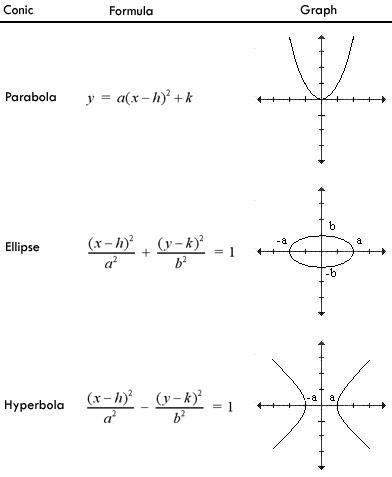The manipulation of conics is similar to the manipulation of circles, where h shifts the conic horizontally, k shifts vertically and these two values, in the form (h,k), are the coordinates of the center or vertex of the conic. The variables a and b control the size and shape of the conic as r does with the circle. Remember that a and b are squared, the same as r is squared in the circle equation. See the note in the Circle Equation section for more details.

The quadratic equation tutorial has a more in depth look at the parabola.

# Examples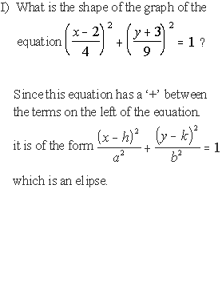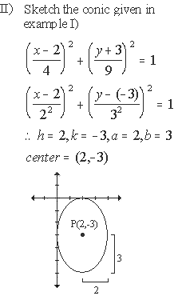1 | Find the volume and surface area of the figure
2 | Find the midpoint and distance between points P and Q

| Top of Page |

COURSE HOMEPAGES
MATH 1036
MATH 1037

FACULTY HOMEPAGES
Alex Karassev
Ted Chase